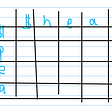# Solution

## 215. Kth Largest Element in an Array

`# O(nlgn) timedef findKthLargest1(self, nums, k):    return sorted(nums, reverse=True)[k-1]    # O(nk) time, bubble sort idea, TLEdef findKthLargest2(self, nums, k):    for i in xrange(k):        for j in xrange(len(nums)-i-1):            if nums[j] > nums[j+1]:                # exchange elements, time consuming                nums[j], nums[j+1] = nums[j+1], nums[j]    return nums[len(nums)-k]    # O(nk) time, selection sort ideadef findKthLargest3(self, nums, k):    for i in xrange(len(nums), len(nums)-k, -1):        tmp = 0        for j in xrange(i):            if nums[j] > nums[tmp]:                tmp = j        nums[tmp], nums[i-1] = nums[i-1], nums[tmp]    return nums[len(nums)-k]    # O(k+(n-k)lgk) time, min-heapdef findKthLargest4(self, nums, k):    heap = []    for num in nums:        heapq.heappush(heap, num)    for _ in xrange(len(nums)-k):        heapq.heappop(heap)    return heapq.heappop(heap)# O(k+(n-k)lgk) time, min-heap        def findKthLargest5(self, nums, k):    return heapq.nlargest(k, nums)[k-1]    # O(n) time, quick selectiondef findKthLargest(self, nums, k):    # convert the kth largest to smallest    return self.findKthSmallest(nums, len(nums)+1-k)    def findKthSmallest(self, nums, k):    if nums:        pos = self.partition(nums, 0, len(nums)-1)        if k > pos+1:            return self.findKthSmallest(nums[pos+1:], k-pos-1)        elif k < pos+1:            return self.findKthSmallest(nums[:pos], k)        else:            return nums[pos] # choose the right-most element as pivot   def partition(self, nums, l, r):    low = l    while l < r:        if nums[l] < nums[r]:            nums[l], nums[low] = nums[low], nums[l]            low += 1        l += 1    nums[low], nums[r] = nums[r], nums[low]    return low`

## When will we get the worst-case complexity for quickselect algorithm

`function partition(list, left, right, pivotIndex) is    pivotValue := list[pivotIndex]    swap list[pivotIndex] and list[right]  // Move pivot to end    storeIndex := left    for i from left to right − 1 do        if list[i] < pivotValue then            swap list[storeIndex] and list[i]            increment storeIndex    swap list[right] and list[storeIndex]  // Move pivot to its final place    return storeIndex// Returns the k-th smallest element of list within left..right inclusive// (i.e. left <= k <= right).function select(list, left, right, k) is    if left = right then   // If the list contains only one element,        return list[left]  // return that element    pivotIndex  := ...     // select a pivotIndex between left and right,                           // e.g., left + floor(rand() % (right − left + 1))    pivotIndex  := partition(list, left, right, pivotIndex)    // The pivot is in its final sorted position    if k = pivotIndex then        return list[k]    else if k < pivotIndex then        return select(list, left, pivotIndex − 1, k)    else        return select(list, pivotIndex + 1, right, k)`

## Direct translate pseudocode to C++ code

`/*Runtime: 16 ms, faster than 95.11% of C++ online submissions for Kth Largest Element in an Array.Memory Usage: 10 MB, less than 96.23% of C++ online submissions for Kth Largest Element in an Array.*/class Solution {public:    int partition(vector<int>& list, int left, int right,int pivot_index)    {        auto pivot_value = list[pivot_index];        swap(list[pivot_index], list[right]);        auto store_index = left;        for(int i=left; i<=right;++i)        {            if (list[i] < pivot_value)            {                swap(list[i], list[store_index++]);            }         }        swap(list[store_index], list[right]);        return store_index;    }        int select(vector<int>& list, int left, int right,int k)    {        if (left==right)return list[left];        auto pivot_index = left + rand()%(right - left + 1);        pivot_index = partition(list, left, right, pivot_index);        if (k==pivot_index)return list[pivot_index];        else if(k<pivot_index)        {             return select(list, left, pivot_index-1, k);        }        else        {            return select(list, pivot_index+1, right, k);        }               }    int findKthLargest(vector<int>& nums, int k)     {        return select(nums, 0, nums.size()-1, nums.size()-k);    }};`

# Reference

--

--

--

## More from Jimmy Shen

Data Scientist/MLE/SWE @takemobi

Love podcasts or audiobooks? Learn on the go with our new app.

## AWS CLI with Educate Account## Nearshore vs. Offshore Software Outsourcing: Which one is the better option?## Accident Lawyer Sandy Utah## Build your Pokédex: Part 3 — Improve NgRX using create* functions## EE5111 Selected Topics in Industrial Control & Instrumentation## Minimum number of deletions and insertions python dynamic programming## Jimmy Shen

Data Scientist/MLE/SWE @takemobi

## Data Structures 101: Introduction to Data Structures and Algorithms